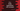# C++ STL find() explanation with example## C++ STL find() explanation with example:

std::find function of C++ STL is used to find a value in a range. In this post, we will learn how to use find with examples.

### Definition of find():

find is defined as below:

``InputIterator find (InputIterator first, InputIterator last, const T& val)``

Here,

• first is the input iterator to the first element in the sequence.
• last is the input iterator to the last element in the sequence.
• val is the value to search in the given range.

It searched for the val in range [first, last) i.e. it searches for all the elements between first to last including the element at position first but not the element at position last.

### C++ program to use find():

Below C++ program shows how to use find:

``````#include <iostream>
#include <algorithm>
using namespace std;

int main()
{
int givenValues[] = {1, 2, 3, 4, 5, 6, 7, 8, 9, 10};
int value;

cout << "Enter a value to search : " << endl;
cin >> value;

int *searchedResult = std::find(givenValues, givenValues + 10, value);

if (searchedResult != givenValues + 10)
cout << "Found : " << *searchedResult << endl;
else
}``````

Here,

• givenValues is an array of integers.
• value is the value to search in the array that we are taking as input from the user.
• Using find, we are searching for the value from start to end of the array. To use this function, we need to import algorithm header.
• Based on its result, we are printing one message that the item is found in the array or not.

### Sample output:

The above program will give output as like below:

``````Enter a value to search :
10
Found : 10

Enter a value to search :
1
Found : 1

Enter a value to search :
11